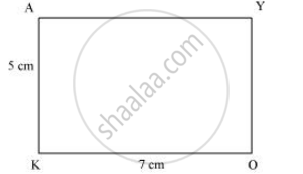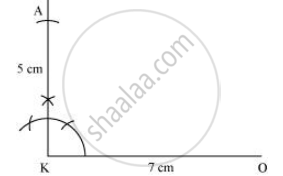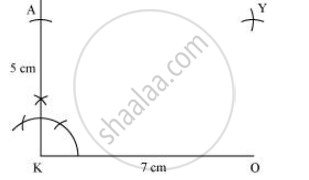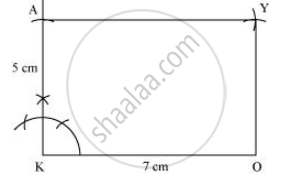Share

# Construct the Following Quadrilaterals. Rectangle Okay Ok = 7 Cm Ka = 5 Cm - CBSE Class 8 - Mathematics

ConceptConstructing a Quadrilateral When Two Adjacent Sides and Three Angles Are Known

Rectangle OKAY

OK = 7 cm

KA = 5 cm

#### Solution

1) A rough sketch of this quadrilateral is drawn as follows.2) Draw a line segment OK of 7 cm and an angle of 90º at point K. As vertex A is 5 cm away from vertex K, cut a line segment KA of 5 cm from this ray.3) Vertex Y is 5 cm and 7 cm away from vertex O and A respectively. By taking radius as 5 cm and 7 cm, draw arcs from point O and A respectively. These will be intersecting each other at point Y.4) Join Y to A and O